# Chapter 2 2 Scientific Notation Scientific Notation Expresses

• Slides: 12Chapter 2. 2 Scientific Notation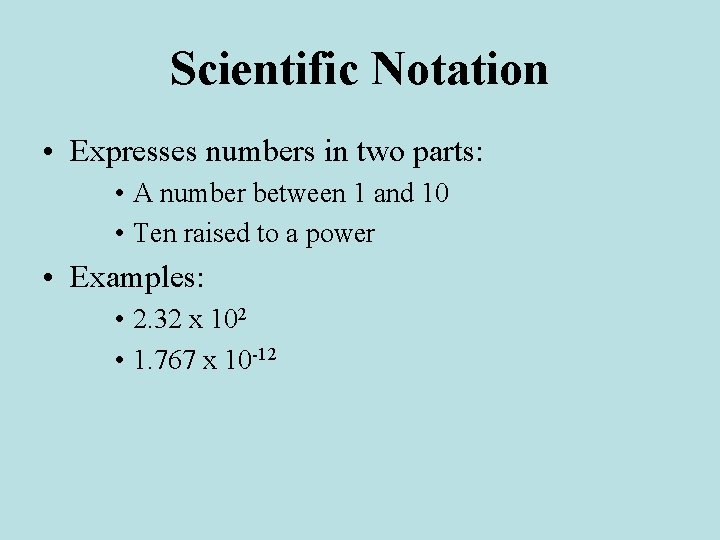Scientific Notation • Expresses numbers in two parts: • A number between 1 and 10 • Ten raised to a power • Examples: • 2. 32 x 102 • 1. 767 x 10 -12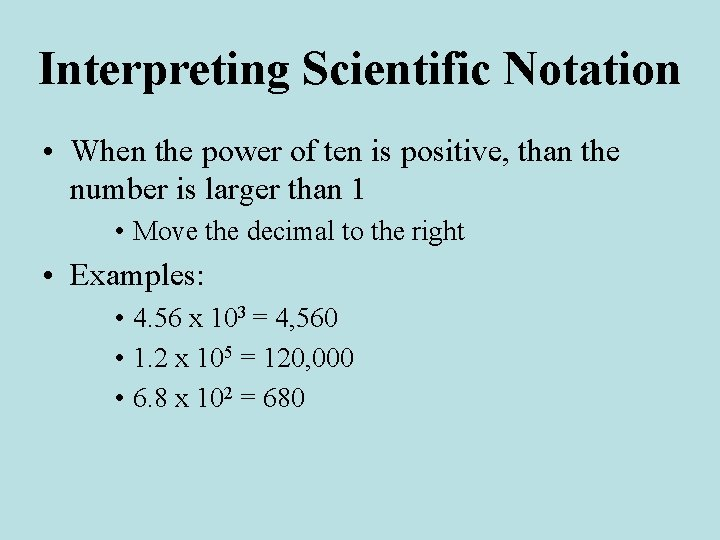Interpreting Scientific Notation • When the power of ten is positive, than the number is larger than 1 • Move the decimal to the right • Examples: • 4. 56 x 103 = 4, 560 • 1. 2 x 105 = 120, 000 • 6. 8 x 102 = 680Interpreting Scientific Notation • When the power of ten is negative, than the number is smaller than 1 • Move the decimal to the left • Examples: • 5. 23 x 10 -3 = 0. 00523 • 2. 03 x 10 -2 = 0. 0203 • 7 x 10 -5 = 0. 000007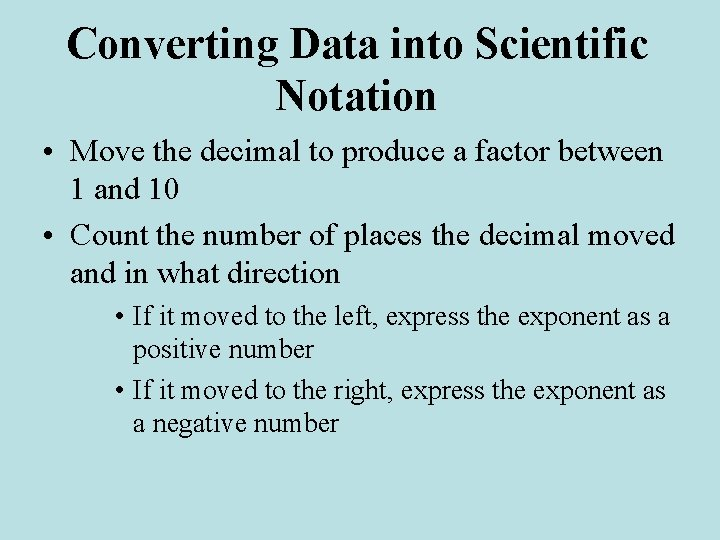Converting Data into Scientific Notation • Move the decimal to produce a factor between 1 and 10 • Count the number of places the decimal moved and in what direction • If it moved to the left, express the exponent as a positive number • If it moved to the right, express the exponent as a negative number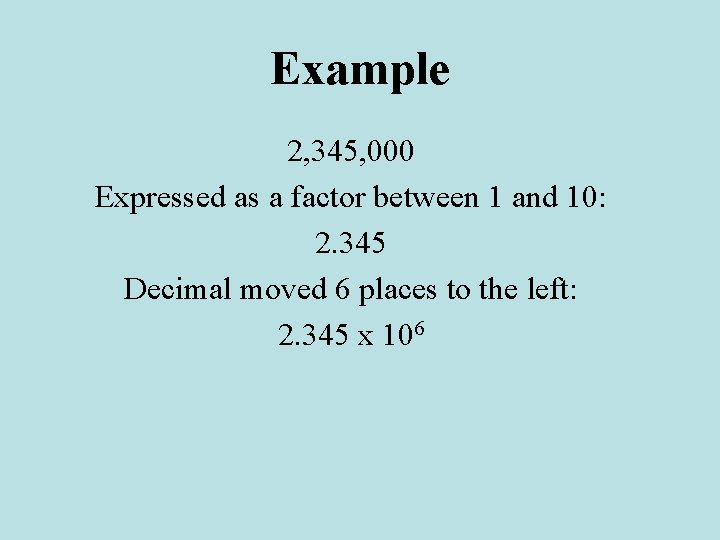Example 2, 345, 000 Expressed as a factor between 1 and 10: 2. 345 Decimal moved 6 places to the left: 2. 345 x 106Example 0. 00178 Expressed as a factor between 1 and 10: 1. 78 Decimal moved 3 places to the right: 1. 78 x 10 -3Adding and Subtracting • Only add and subtract numbers with the same exponent • Convert numbers to the same power of ten before adding or subtracting 6 x 102 + 3 x 103 = 6 x 102 + 30 x 102 = 36 x 102 = 3. 6 x 103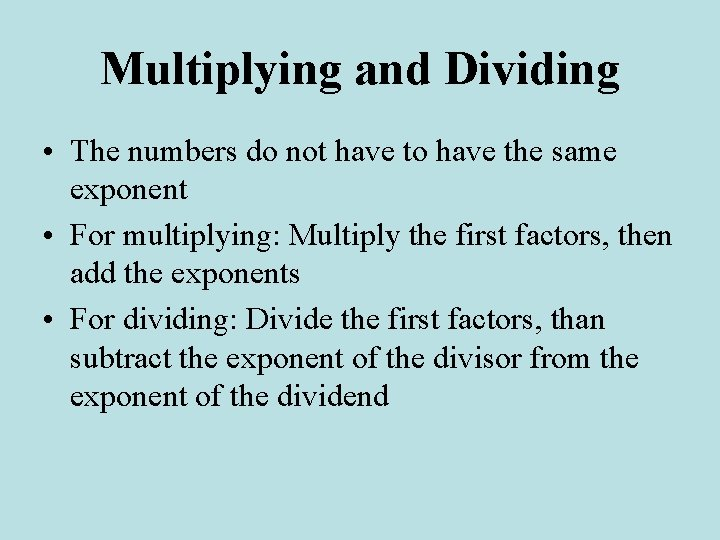Multiplying and Dividing • The numbers do not have to have the same exponent • For multiplying: Multiply the first factors, then add the exponents • For dividing: Divide the first factors, than subtract the exponent of the divisor from the exponent of the dividend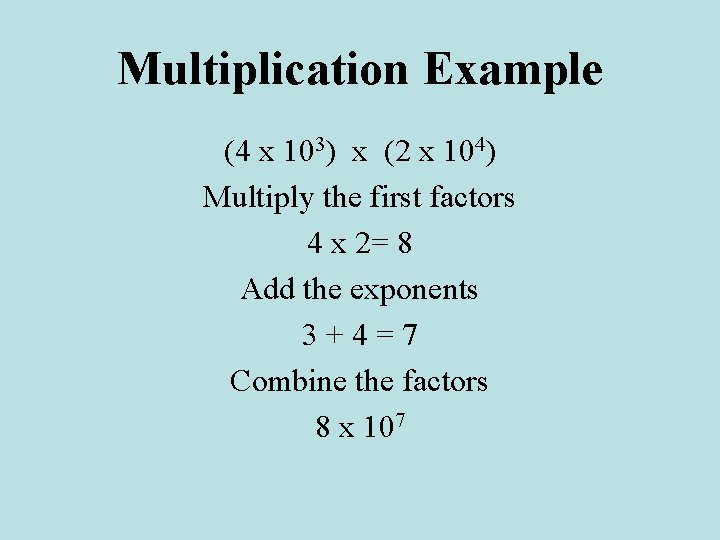Multiplication Example (4 x 103) x (2 x 104) Multiply the first factors 4 x 2= 8 Add the exponents 3+4=7 Combine the factors 8 x 107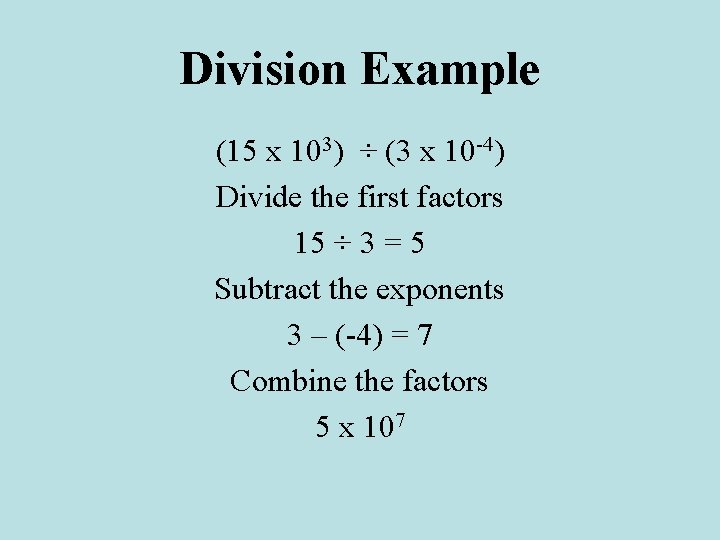Division Example (15 x 103) ÷ (3 x 10 -4) Divide the first factors 15 ÷ 3 = 5 Subtract the exponents 3 – (-4) = 7 Combine the factors 5 x 107Homework • Practice problems 12 -21 on pages 32 -35